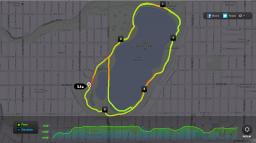# Passenger 1810

The distance between cities A and B is 165 km. A truck traveled from city A to city B at a speed of 50 km/h. Fifteen minutes later, a passenger drove from city B to city A at 72 km/h. How far will the passenger car travel before the two cars meet?

s =  90 km

### Step-by-step explanation:

50(t+15/60) + 72 t = 165
s = 72 t

50·(t+15/60) + 72·t = 165
s = 72·t

7320t = 9150
s-72t = 0

Pivot: Row 1 ↔ Row 2
s-72t = 0
7320t = 9150

t = 9150/7320 = 1.25
s = 0+72t = 0+72 · 1.25 = 90

s = 90
t = 5/4 = 1.25

Our linear equations calculator calculates it.Did you find an error or inaccuracy? Feel free to write us. Thank you!

Tips for related online calculators
Do you want to convert velocity (speed) units?
Do you want to convert time units like minutes to seconds?